# Resources tagged with: Generalising

Filter by: Content type:
Age range:
Challenge level:

### There are 140 results### Mini-max

##### Age 11 to 14 Challenge Level:

Consider all two digit numbers (10, 11, . . . ,99). In writing down all these numbers, which digits occur least often, and which occur most often ? What about three digit numbers, four digit numbers. . . .### Chocolate Maths

##### Age 11 to 14 Challenge Level:

Pick the number of times a week that you eat chocolate. This number must be more than one but less than ten. Multiply this number by 2. Add 5 (for Sunday). Multiply by 50... Can you explain why it. . . .##### Age 11 to 14 Challenge Level:

Think of a number, add one, double it, take away 3, add the number you first thought of, add 7, divide by 3 and take away the number you first thought of. You should now be left with 2. How do I. . . .### Repeaters

##### Age 11 to 14 Challenge Level:

Choose any 3 digits and make a 6 digit number by repeating the 3 digits in the same order (e.g. 594594). Explain why whatever digits you choose the number will always be divisible by 7, 11 and 13.### Three Times Seven

##### Age 11 to 14 Challenge Level:

A three digit number abc is always divisible by 7 when 2a+3b+c is divisible by 7. Why?##### Age 11 to 14 Challenge Level:

List any 3 numbers. It is always possible to find a subset of adjacent numbers that add up to a multiple of 3. Can you explain why and prove it?##### Age 11 to 14 Challenge Level:

A little bit of algebra explains this 'magic'. Ask a friend to pick 3 consecutive numbers and to tell you a multiple of 3. Then ask them to add the four numbers and multiply by 67, and to tell you. . . .### Summing Consecutive Numbers

##### Age 11 to 14 Challenge Level:

15 = 7 + 8 and 10 = 1 + 2 + 3 + 4. Can you say which numbers can be expressed as the sum of two or more consecutive integers?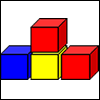### Up and Down Staircases

##### Age 7 to 11 Challenge Level:

One block is needed to make an up-and-down staircase, with one step up and one step down. How many blocks would be needed to build an up-and-down staircase with 5 steps up and 5 steps down?### Window Frames

##### Age 5 to 14 Challenge Level:

This task encourages you to investigate the number of edging pieces and panes in different sized windows.### Special Sums and Products

##### Age 11 to 14 Challenge Level:

Find some examples of pairs of numbers such that their sum is a factor of their product. eg. 4 + 12 = 16 and 4 × 12 = 48 and 16 is a factor of 48.### Sum Equals Product

##### Age 11 to 14 Challenge Level:

The sum of the numbers 4 and 1 [1/3] is the same as the product of 4 and 1 [1/3]; that is to say 4 + 1 [1/3] = 4 � 1 [1/3]. What other numbers have the sum equal to the product and can this be so for. . . .### One O Five

##### Age 11 to 14 Challenge Level:

You can work out the number someone else is thinking of as follows. Ask a friend to think of any natural number less than 100. Then ask them to tell you the remainders when this number is divided by. . . .### Card Trick 2

##### Age 11 to 14 Challenge Level:

Can you explain how this card trick works?##### Age 7 to 14 Challenge Level:

I added together some of my neighbours' house numbers. Can you explain the patterns I noticed?### Have You Got It?

##### Age 11 to 14 Challenge Level:

Can you explain the strategy for winning this game with any target?### Magic Letters

##### Age 11 to 14 Challenge Level:

Charlie has made a Magic V. Can you use his example to make some more? And how about Magic Ls, Ns and Ws?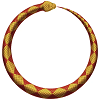### Snake Coils

##### Age 7 to 11 Challenge Level:

This challenge asks you to imagine a snake coiling on itself.### Regular Hexagon Loops

##### Age 11 to 14 Challenge Level:

Make some loops out of regular hexagons. What rules can you discover?### Round the Four Dice

##### Age 7 to 11 Challenge Level:

This activity involves rounding four-digit numbers to the nearest thousand.### Always, Sometimes or Never?

##### Age 5 to 11 Challenge Level:

Are these statements relating to odd and even numbers always true, sometimes true or never true?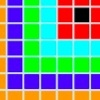### Walking the Squares

##### Age 7 to 11 Challenge Level:

Find a route from the outside to the inside of this square, stepping on as many tiles as possible.### Always, Sometimes or Never? Number

##### Age 7 to 11 Challenge Level:

Are these statements always true, sometimes true or never true?### Enclosing Squares

##### Age 11 to 14 Challenge Level:

Can you find sets of sloping lines that enclose a square?### Odd Squares

##### Age 7 to 11 Challenge Level:

Think of a number, square it and subtract your starting number. Is the number you’re left with odd or even? How do the images help to explain this?### Go Forth and Generalise

##### Age 11 to 14

Spotting patterns can be an important first step - explaining why it is appropriate to generalise is the next step, and often the most interesting and important.### Number Differences

##### Age 7 to 11 Challenge Level:

Place the numbers from 1 to 9 in the squares below so that the difference between joined squares is odd. How many different ways can you do this?### Crossings

##### Age 7 to 11 Challenge Level:

In this problem we are looking at sets of parallel sticks that cross each other. What is the least number of crossings you can make? And the greatest?### Keep it Simple

##### Age 11 to 14 Challenge Level:

Can all unit fractions be written as the sum of two unit fractions?### More Number Pyramids

##### Age 11 to 14 Challenge Level:

When number pyramids have a sequence on the bottom layer, some interesting patterns emerge...### What Numbers Can We Make?

##### Age 11 to 14 Challenge Level:

Imagine we have four bags containing a large number of 1s, 4s, 7s and 10s. What numbers can we make?### Truth or Lie

##### Age 7 to 11 Challenge Level:

Take a look at the video of this trick. Can you perform it yourself? Why is this maths and not magic?### Got it for Two

##### Age 7 to 14 Challenge Level:

Got It game for an adult and child. How can you play so that you know you will always win?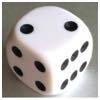### Roll over the Dice

##### Age 7 to 11 Challenge Level:

Watch this video to see how to roll the dice. Now it's your turn! What do you notice about the dice numbers you have recorded?### Round the Three Dice

##### Age 7 to 11 Challenge Level:

What happens when you round these three-digit numbers to the nearest 100?### What Numbers Can We Make Now?

##### Age 11 to 14 Challenge Level:

Imagine we have four bags containing numbers from a sequence. What numbers can we make now?##### Age 5 to 11 Challenge Level:

Try out this number trick. What happens with different starting numbers? What do you notice?### Three Dice

##### Age 7 to 11 Challenge Level:

Investigate the sum of the numbers on the top and bottom faces of a line of three dice. What do you notice?### Partitioning Revisited

##### Age 11 to 14 Challenge Level:

We can show that (x + 1)² = x² + 2x + 1 by considering the area of an (x + 1) by (x + 1) square. Show in a similar way that (x + 2)² = x² + 4x + 4### Surprising Split

##### Age 7 to 11 Challenge Level:

Does this 'trick' for calculating multiples of 11 always work? Why or why not?### Handshakes

##### Age 11 to 14 Challenge Level:

Can you find an efficient method to work out how many handshakes there would be if hundreds of people met?### Egyptian Fractions

##### Age 11 to 14 Challenge Level:

The Egyptians expressed all fractions as the sum of different unit fractions. Here is a chance to explore how they could have written different fractions.### Elevenses

##### Age 11 to 14 Challenge Level:

How many pairs of numbers can you find that add up to a multiple of 11? Do you notice anything interesting about your results?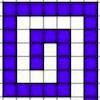### Spirals, Spirals

##### Age 7 to 11 Challenge Level:

Here are two kinds of spirals for you to explore. What do you notice?### Consecutive Negative Numbers

##### Age 11 to 14 Challenge Level:

Do you notice anything about the solutions when you add and/or subtract consecutive negative numbers?### Finding 3D Stacks

##### Age 7 to 11 Challenge Level:

Can you find a way of counting the spheres in these arrangements?### Number Pyramids

##### Age 11 to 14 Challenge Level:

Try entering different sets of numbers in the number pyramids. How does the total at the top change?### More Magic Potting Sheds

##### Age 11 to 14 Challenge Level:

The number of plants in Mr McGregor's magic potting shed increases overnight. He'd like to put the same number of plants in each of his gardens, planting one garden each day. How can he do it?### Nim-7

##### Age 5 to 14 Challenge Level:

Can you work out how to win this game of Nim? Does it matter if you go first or second?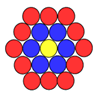### Counting Counters

##### Age 7 to 11 Challenge Level:

Take a counter and surround it by a ring of other counters that MUST touch two others. How many are needed?